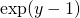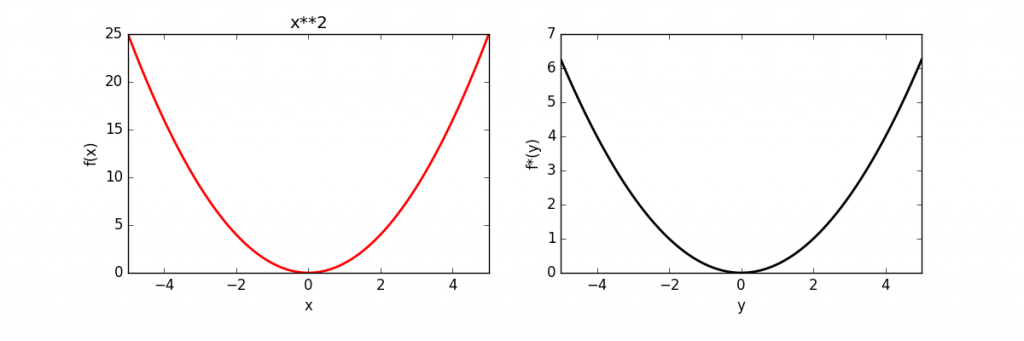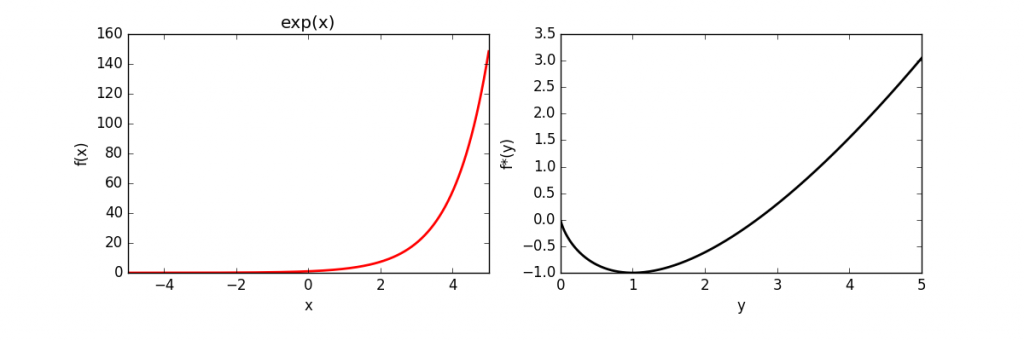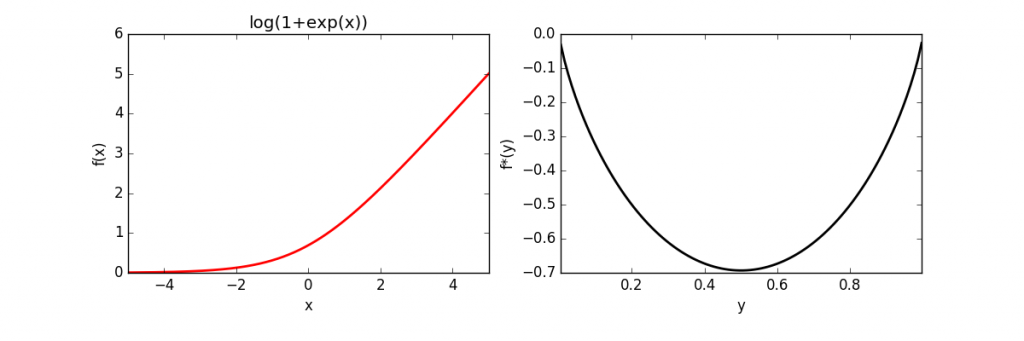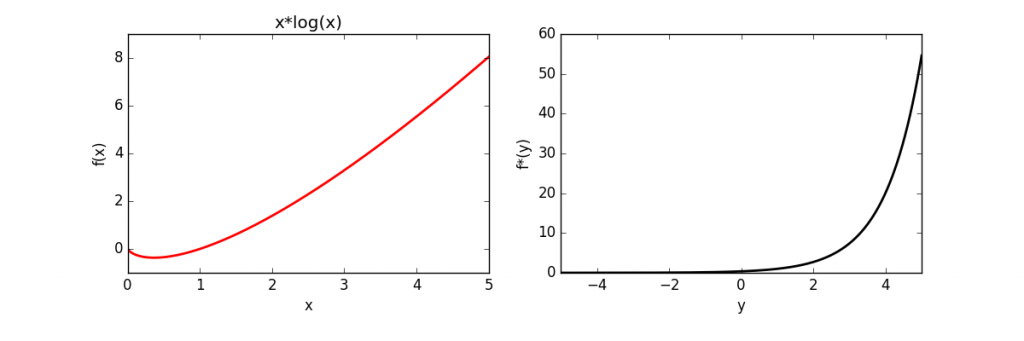## Automatically calculating the convex conjugate (Fenchel dual) using Python/Sympy

The convex conjugate (or Fenchel dual) for a single variable function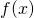is defined as: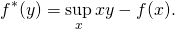We assume thatis convex, differentiable and simple (closed-form solution exist for minimum). The conjugate can then symbolically be calculated using the following Python code:

def calc_conjugate(str, varnames='x'):

# set the symbols
vars = sp.symbols(varnames)
x = vars if isinstance(vars, tuple) else vars
y = sp.symbols('y', real=True)

# set the function and objective
fun = parse_expr(str)
obj = x*y - fun

# calculate derivative of obj and solve for zero
sol = solve(sp.diff(obj, x), x)

# substitute solution into objective
solfun = sp.simplify(obj.subs(x, sol))

# if extra values were passed add to lambda function
varnames = [y] + list(vars[1:]) if isinstance(vars, tuple) else y

return (sp.sstr(solfun), lambdify(vars, fun, 'numpy'), lambdify(varnames, solfun, 'numpy'))


The full source code is here. The above code was used to calculate and plot the conjugates of several functions, which is given below.

 Function Conjugate Quadratic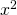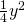Exponential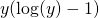Log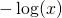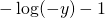Log-Exponential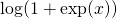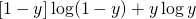x log(x)